# Flow in pipes

## Pipe diameter, Bernoulli equation, pressure drop, friction factor### Fluid flow mean velocity and pipe diameter for known flow rate

Velocity of fluid in pipe is not uniform across section area. Therefore a mean velocity is used and it is calculated by the continuity equation for the steady flow as:### Pipe diameter calculator

Calculate pipe diameter for known flow rate and velocity. Calculate flow velocity for known pipe diameter and flow rate. Convert from volumetric to mass flow rate. Calculate volumetric flow rate of ideal gas at different conditions of pressure and temperature.

Pipe diameter can be calculated when volumetric flow rate and velocity is known as: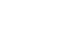where is: D - internal pipe diameter; q - volumetric flow rate; v - velocity; A - pipe cross section area.

If mass flow rate is known than diameter can be calculated as: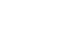where is: D - internal pipe diameter; w - mass flow rate; ρ - fluid density; v - velocity.

### Laminar and turbulent fluid flow regime in pipe, critical velocity

If the velocity of fluid inside the pipe is small, streamlines will be in straight parallel lines. As the velocity of fluid inside the pipe gradually increase, streamlines will continue to be straight and parallel with the pipe wall until velocity is reached when the streamlines will waver and suddenly break into diffused patterns. The velocity at which this occurs is called "critical velocity". At velocities higher than "critical", the streamlines are dispersed at random throughout the pipe.

The regime of flow when velocity is lower than "critical" is called laminar flow (or viscous or streamline flow). At laminar regime of flow the velocity is highest on the pipe axis, and on the wall the velocity is equal to zero.

When the velocity is greater than "critical", the regime of flow is turbulent. In turbulent regime of flow there is irregular random motion of fluid particles in directions transverse to the direction on main flow. Velocity change in turbulent flow is more uniform than in laminar.

In the turbulent regime of flow, there is always a thin layer of fluid at pipe wall which is moving in laminar flow. That layer is known as the boundary layer or laminar sub-layer. To determine flow regime use Reynolds number calculator.

### Reynolds number, turbulent and laminar flow, pipe flow velocity and viscosity

The nature of flow in pipe, by the work of Osborne Reynolds, is depending on the pipe diameter, the density and viscosity of the flowing fluid and the velocity of the flow. Dimensionless Reynolds number is used, and is combination of these four variables and may be considered to be ratio of dynamic forces of mass flow to the shear stress due to viscosity. Reynolds number is:where is: D - internal pipe diameter; v - velocity; ρ - density; ν - kinematic viscosity; μ - dynamic viscosity;

### Reynolds number calculator

Calculate Reynolds number with this easy to use calculator. Determine if flow is laminar or turbulent. Applicable for liquids and gases.

This equation can be solved using and fluid flow regime calculator.

Flow in pipes is considered to be laminar if Reynolds number is less than 2320, and turbulent if the Reynolds number is greater than 4000. Between these two values is "critical" zone where the flow can be laminar or turbulent or in the process of change and is mainly unpredictable.

When calculating Reynolds number for non-circular cross section equivalent diameter (four time hydraulic radius d=4xRh) is used and hydraulic radius can be calculated as:

Rh = cross section flow area / wetted perimeter

It applies to square, rectangular, oval or circular conduit when not flowing with full section. Because of great variety of fluids being handled in modern industrial processes, a single equation which can be used for the flow of any fluid in pipe offers big advantages. That equation is Darcy formula, but one factor - the friction factor has to be determined experimentally. This formula has a wide application in the field of fluid mechanics and is used extensively throughout on this web site.

### Bernoulli equation - fluid flow head conservation

If friction losses are neglected and no energy is added to, or taken from a piping system, the total head, H, which is the sum of the elevation head, the pressure head and the velocity head will be constant for any point of fluid streamline.

This is the expression of law of head conservation to the flow of fluid in a conduit or streamline and is known as Bernoulli equation:where is: Z1,2 - elevation above reference level; p1,2 - absolute pressure; v1,2 - velocity; ρ1,2 - density; g - acceleration of gravity

Bernoulli equation equation is used in several calculators on this site like pressure drop and flow rate calculator, Venturi tube flow rate meter and Venturi effect calculator and orifice plate sizing and flow rate calculator.

### Pipe flow and friction pressure drop, head energy loss | Darcy formula

From Bernoulli equation all other practical formulas are derived, with modifications due to energy losses and gains.

As in real piping system, losses of energy are existing and energy is being added to or taken from the fluid (using pumps and turbines) these must be included in the Bernoulli equation.

For two points of one streamline in a fluid flow, equation may be written as follows:where is: Z1,2 - elevation above reference level; p1,2 - absolute pressure; v1,2 - velocity; ρ1,2 - density; hL - head loss due to friction in the pipe; Hp - pump head; HT - turbine head; g - acceleration of gravity;

Flow in pipe is always creating energy loss due to friction. Energy loss can be measured like static pressure drop in the direction of fluid flow with two gauges. General equation for pressure drop, known as Darcy's formula expressed in meters of fluid is:where is: hL - head loss due to friction in the pipe; f - friction coefficient; L - pipe length; v - velocity; D - internal pipe diameter; g - acceleration of gravity;

To express this equation like pressure drop in newtons per square meter (Pascals) substitution of proper units leads to:### Pressure drop calculator

Calculator based on Darcy equation. Calculate pressure drop for known flow rate or calculate flow rate for known pressure drop. Friction factor calculation included. Applicable for laminar and turbulent flow, circular or rectangle pipe.

where is: Δ p - pressure drop due to friction in the pipe; ρ - density; f - friction coefficient; L - pipe length; v - velocity; D - internal pipe diameter; Q - volumetric flow rate;

The Darcy equation can be used for both laminar and turbulent flow regime and for any liquid in a pipe. With some restrictions, Darcy equation can be used for gases and vapors. Darcy formula applies when pipe diameter and fluid density is constant and the pipe is relatively straight.

### Friction factor for pipe roughness and Reynolds number in laminar and turbulent flow

Physical values in Darcy formula are very obvious and can be easily obtained when pipe properties are known like D - pipe internal diameter, L - pipe length and when flow rate is known, velocity can be easily calculated using continuity equation. The only value that needs to be determined experimentally is friction factor. For laminar flow regime Re < 2000, friction factor can be calculated, but for turbulent flow regime where is Re > 4000 experimentally obtained results are used. In the critical zone, where is Reynolds number between 2000 and 4000, both laminar and turbulent flow regime might occur, so friction factor is indeterminate and has lower limits for laminar flow, and upper limits based on turbulent flow conditions.

If the flow is laminar and Reynolds number is smaller than 2000, the friction factor may be determined from the equation:where is: f - friction factor; Re - Reynolds number;

When flow is turbulent and Reynolds number is higher than 4000, the friction factor depends on pipe relative roughness as well as on the Reynolds number. Relative pipe roughness is the roughness of the pipe wall compared to pipe diameter e/D. Since the internal pipe roughness is actually independent of pipe diameter, pipes with smaller pipe diameter will have higher relative roughness than pipes with bigger diameter and therefore pipes with smaller diameters will have higher friction factors than pipes with bigger diameters of the same material.

Most widely accepted and used data for friction factor in Darcy formula is the Moody diagram. On Moody diagram friction factor can be determined based on the value of Reynolds number and relative roughness.

The pressure drop is the function of internal diameter with the fifth power. With time in service, the interior of the pipe becomes encrusted with dirt, scale and it is often prudent to make allowance for expected diameter changes. Also roughness may be expected to increase with use due to corrosion or incrustation at a rate determined by the pipe material and nature of the fluid.

When the thickness of laminar sub layer (laminar boundary layer δ) is bigger than the pipe roughness e the flow is called flow in hydraulically smooth pipe and Blasius equation can be used: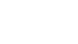where is: f - friction factor; Re - Reynolds number;

The boundary layer thickness can be calculated based on the Prandtl equation as:where is: δ - boundary layer thickness; D - internal pipe diameter; Re - Reynolds number;

For turbulent flow with Re < 100 000 (Prandtl equation) can be used:For turbulent flow with Re > 100 000 (Karman equation) can be used: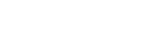Most common equation used for friction coefficient calculation is Colebrook-White formula and it is used for the turbulent flow in the pressure drop calculator:where is: f - friction factor; Re - Reynolds number; D - internal pipe diameter; kr - pipe roughness;

### Static, dynamic and total pressure, flow velocity and Mach number

Static pressure is pressure of fluid in flow stream. Total pressure is pressure of fluid when it is brought to rest, i.e. velocity is reduced to 0.

Total pressure can be calculated using Bernoulli theorem. Imagining that flow is in one point of stream line stopped without any energy loss Bernoulli theorem can be written as:If velocity at point 2 v2=0, pressure at point 2 is than total p2=pt:where is: p - pressure; pt - total pressure; v - velocity; ρ - density;

The difference between total and static pressure represents fluid kinetic energy and it is called dynamic pressure.

Dynamic pressure for liquids and incompressible flow where the density is constant can be calculated as: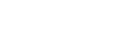where is: p - pressure; pt - total pressure; pd - dynamic pressure; v - velocity; ρ - density;

If dynamic pressure is measured using instruments like Prandtl probe or Pitot tube velocity can be calculated in one point of stream line as:where is: p - pressure; pt - total pressure; pd - dynamic pressure; v - velocity; ρ - density;

For gases and larger Mach numbers than 0.1 effects of compressibility are not negligible.

For compressible flow calculation gas state equation can be used. For ideal gases, velocity for Mach number M < 1 is calculated using following equation:where is: M - Mach number M=v/c - relation between local fluid and local sound velocity; γ - isentropic coefficient;

It should be said that for M > 0.7 given equation is not totally accurate.

If Mach number M > 1, than normal shock wave will occur. Equation for velocity in front of the wave is given bellow:where is: p - pressure; pti - total pressure; v - velocity; M - Mach number; γ - isentropic coefficient;

Above equations are used for Prandtl probe and Pitot tube flow velocity calculator.

Note: You can download complete derivation of given equations

### Thermal energy calculator

Calculate heat energy and thermal power for known flow rate. Calculate flow rate for known heat energy or thermal power. Applicable for boilers, heat exchangers, radiators, chillers, air heaters.

The flow rate of fluid required for the thermal energy - heat power transfer can be calculated as:where is: q - flow rate [m3/h]; ρ - density of fluid [kg/m3]; c - specific heat of fluid [kJ/kgK]; Δ T - temperature difference [K]; P - power [kW];

This relation can be used to calculate required flow rate of, for example, water heated in the boiler, if the power of boiler is known. In that case temperature difference in above equation is the change of temperature of fluid in front and after the boiler. It should be said that efficiency coefficient should be included in above equation, for precise calculation.

• Pipe flow theory pages
• Fluid properties
Fluid density, specific volume, specific gravity, mixture density, viscosity, pressure levels
• Incompressible flow in pipe
Pipe diameter, Reynolds number, laminar/turbulent flow, Darcy formula, friction factor, dynamic/total pressure, heat energy
• Flow in valves and fittings
Resistance coefficient, flow coefficient, cavitation, chocked flow, flow discharge, gas regulator, natural gas control valves
• Compressible flow
Adiabatic, isothermal flow, fuel combustion, maximum flow, evaporation energy